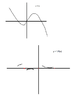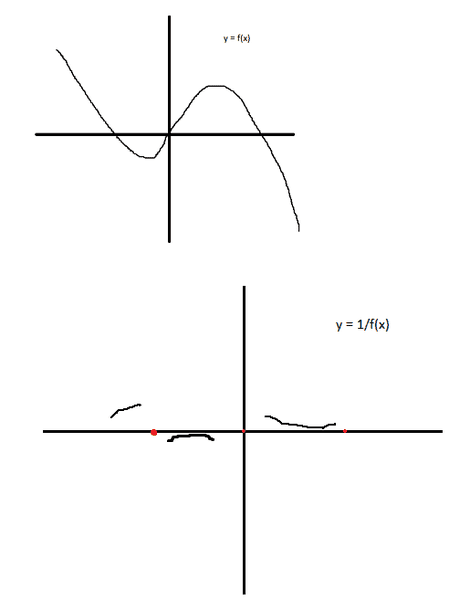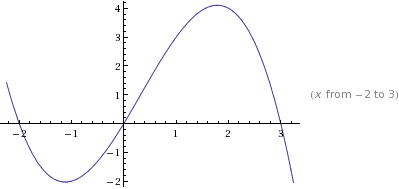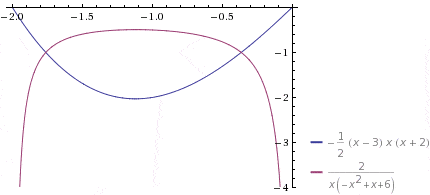# Not sure if I'm drawing the reciprocal function properly

priceofcarrot

## Homework Statement

The y = f(x) is the original graph. The question asks me to draw the y = 1/f(x) of that graph. What I did was just use the same x coordinates and then take the y coordinates and just do 1/y coordinate for each y coordinate. Is this the correct way to do it?

Also, the red dots on the graph of the y = 1/f(x) are the areas of the graph that are undefined (meaning I couldn't do 1/y coordinate, because the y coordinate was 0). Does that mean that those points are asymptotes? If they are, how do I figure out if they're horizontal or vertical asymptotes?

Also, you'll see that right before and after the red dots on the graph of y = 1/f(x) there is empty space. I wasn't sure if I was supposed to draw the lines of the graph up until the red dots (the asymptotes if that is what they represent), or just until the points that were available to me (the ones that I got from the original graph and then did 1/by the y coordinates).

Thanks

EDIT: Those aren't my final graphs. They're just quick drawings to illustrate what I'm talking about.

## The Attempt at a Solution

#### Attachments

•physicsforumimagegraph.png
4.7 KB · Views: 660

Staff Emeritus
Homework Helper
Gold Member

## Homework Statement

The y = f(x) is the original graph. The question asks me to draw the y = 1/f(x) of that graph. What I did was just use the same x coordinates and then take the y coordinates and just do 1/y coordinate for each y coordinate. Is this the correct way to do it?

Also, the red dots on the graph of the y = 1/f(x) are the areas of the graph that are undefined (meaning I couldn't do 1/y coordinate, because the y coordinate was 0). Does that mean that those points are asymptotes? If they are, how do I figure out if they're horizontal or vertical asymptotes?

Also, you'll see that right before and after the red dots The on the graph of y = 1/f(x) there is empty space. I wasn't sure if I was supposed to draw the lines of the graph up until the red dots (the asymptotes if that is what they represent), or just until the points that were available to me (the ones that I got from the original graph and then did 1/by the y coordinates).

Thanks

EDIT: Those aren't my final graphs. They're just quick drawings to illustrate what I'm talking about.The portions you drew to this point are OK.

You should draw in the vertical asymptotes (at the red dots) and show how the graph approaches them.

What is the reciprocal of a very small positive number?

What is the reciprocal of a negative number that's very near zero?

Mentor

## Homework Statement

The y = f(x) is the original graph. The question asks me to draw the y = 1/f(x) of that graph. What I did was just use the same x coordinates and then take the y coordinates and just do 1/y coordinate for each y coordinate. Is this the correct way to do it?
Yes, if I understand what you're trying to say (but not saying very well). If (x, y) is a point on the original graph, the corresponding point on the graph of the reciprocal function will be (x, 1/y).
Also, the red dots on the graph of the y = 1/f(x) are the areas of the graph that are undefined (meaning I couldn't do 1/y coordinate, because the y coordinate was 0). Does that mean that those points are asymptotes? If they are, how do I figure out if they're horizontal or vertical asymptotes?
Let's call the points where you have the red dots A, B, and C, going left to right. Each of these points is an x-intercept for the graph of f. Near each of these points on the graph of f, the y values are close to zero. When you take the reciprocal of a number that's close to zero, what happens?

At the left and right ends of your graph of f, the y values are getting very large for very negative x, and getting very small (very negative) for very large x. What will the y values on the graph of 1/f be doing?

Also, you'll see that right before and after the red dots on the graph of y = 1/f(x) there is empty space. I wasn't sure if I was supposed to draw the lines of the graph up until the red dots (the asymptotes if that is what they represent), or just until the points that were available to me (the ones that I got from the original graph and then did 1/by the y coordinates).

Thanks

EDIT: Those aren't my final graphs. They're just quick drawings to illustrate what I'm talking about.

priceofcarrot
I just noticed something. Those three dots are at y = 0, because y is undefined for 1/0. Does that mean that it's just one horizontal asymptote at y = 0? Not three vertical asymptotes?

What is the reciprocal of a very small positive number?

What is the reciprocal of a negative number that's very near zero?

The reciprocal of a very small positive number is a larger positive number.

The reciprocal of a negative number that's very near zero is a larger negative number.

Let's call the points where you have the red dots A, B, and C, going left to right. Each of these points is an x-intercept for the graph of f. Near each of these points on the graph of f, the y values are close to zero. When you take the reciprocal of a number that's close to zero, what happens?

At the left and right ends of your graph of f, the y values are getting very large for very negative x, and getting very small (very negative) for very large x. What will the y values on the graph of 1/f be doing?

They will be getting larger positive, and larger negative (or smaller negative, I'm not sure if you'd refer to it as smaller or larger negative).

Last edited:
Staff Emeritus
Homework Helper
Gold Member
I just noticed something. Those three dots are at y = 0, because y is undefined for 1/0. Does that mean that it's just one horizontal asymptote? Not three vertical asymptotes?
...
In the given graph, tho only places that y = 0 are at the locations of the red dots. (I already indicated that those are the locations of vertical asymptotes on the graph of the reciprocal function.)

You correctly answered the questions about the reciprocals of positive & negative numbers which are close to zero. Use that information to fill in more of your graph.

priceofcarrot
I recognize that you said that, but I don't understand why that's how it works. I thought that when y is undefined then it's a horizontal asymptote.

Homework Helper
I just noticed something. Those three dots are at y = 0, because y is undefined for 1/0. Does that mean that it's just one horizontal asymptote at y = 0? Not three vertical asymptotes?

I can see why you'd think that, but no, it's not true. There is a horizontal asymptote at y=0 however! But that's only because as x gets very large positvely or negatively ($x\to\pm\infty$), y=1/f(x) approaches zero.

At the finite values of x where the red dots are located however, you cannot take the reciprocal of 0 which is 1/0 so you'll have a vertical asymptote at every point where the graph of f(x) equals to zero.

priceofcarrot
I can see why you'd think that, but no, it's not true. There is a horizontal asymptote at y=0 however! But that's only because as x gets very large positvely or negatively ($x\to\pm\infty$), y=1/f(x) approaches zero.

At the finite values of x where the red dots are located however, you cannot take the reciprocal of 0 which is 1/0 so you'll have a vertical asymptote at every point where the graph of f(x) equals to zero.
Okay, now I'm confused about something else.

You said,
But that's only because as x gets very large positvely or negatively (x→±∞), y=1/f(x) approaches zero.

I don't understand how then in my graph of 1/f(x) on that curve part of the graph that points toward the top, and is positioned to the left of the y axis, right below the x axis, as x goes towards 0, y goes away from 0, but as x goes away from, y goes towards 0, but then also goes away from 0.

I'm referring to the shape of that curve. It starts from the right or left (however you want to look at it) away from 0, and then gets closer to 0 at the middle, but then goes away from 0 again for y, whether x is larger or smaller.

I included a modified version of the original image that might help.

#### Attachments

Staff Emeritus
Homework Helper
Gold MemberThe above is a graph of y=f(x), for f(x) = -x(x+1)(x-2)/2 which looks something like your f(x).

Below is a graph of y=f(x) and y=1/f(x) from x=-2 to x=0.#### Attachments

Homework Helper
Thanks for the image Sammy, hopefully that'll get us all closer to the same page.

priceofcarrot
So 1/f(x) is just a representation, or what they call a reciprocal function?

It's not as if all reciprocal functions are actually 1 / f(x)? It can be 2 / f(x) like in your bottom graph?

I'm still confused about the "But that's only because as x gets very large positvely or negatively (x→±∞), y=1/f(x) approaches zero." part though.

Because to me the reciprocal function in your graph approaches 0 when x becomes a larger negative value, or a smaller negative value. It also distances itself from 0 when x becomes a larger or smaller negative value.

Also, for the initial graph of 1/f(x) that I included, I'm not supposed to use graphing software to draw it. So I have to do it by hand. How do I figure out how far to draw the different parts of the graph?

Last edited:
Mentor
So 1/f(x) is just a representation, or what they call a reciprocal function?
1/f(x) is the reciprocal of f(x).
It's not as if all reciprocal functions are actually 1 / f(x)? It can be 2 / f(x) like in your bottom graph?
No, 2/f(x) is not the reciprocal of f(x).

If you look carefully, you'll see that SammyS's f(x) is (1/2)(x - 3)(x)(x + 2), so the reciprocal (1/f(x)) is 2/[(x - 3)(x)(x + 2)]. The 2 here came from a denominator of 2 in f(x).
I'm still confused about the "But that's only because as x gets very large positvely or negatively (x→±∞), y=1/f(x) approaches zero." part though.

Because to me the reciprocal function in your graph approaches 0 when x becomes a larger negative value, or a smaller negative value. It also distances itself from 0 when x becomes a larger or smaller negative value.
I'm not sure whom you're responding to here.
If the graph of the reciprocal function is approaching 0 for large x or very negative x, it must be true that f(x) is approaching ∞ or -∞ as x gets large or as x gets very negative.
Also, for the initial graph of 1/f(x) that I included, I'm not supposed to use graphing software to draw it. So I have to do it by hand. How do I figure out how far to draw the different parts of the graph?

In your grah of f(x), as x → -∞, f(x) → +∞, so the graph of 1/f(x) will approach 0 (but from above). Can you use similar reasoning to figure out what the graph of 1/f(x) does as x → +∞?

priceofcarrot
Sorry guys I'm confused. To me my graph f(x) goes up and down on both sides, so I don't see how x → -∞, f(x) → +∞ is true.

Mentor
Sorry guys I'm confused. To me my graph f(x) goes up and down on both sides, so I don't see how x → -∞, f(x) → +∞ is true.

Look at the graph of f(x) in post #1, and answer the following questions.

1. As x → -∞, f(x) → ?
2. As x → +∞, f(x) → ?

priceofcarrot
Maybe I don't understand what the symbols mean.

I understand "As x → -∞, f(x) → ?" to mean that as the values of x get larger negative (to the left), then the y value of the graph becomes what? Is that your question?

In my graph though, the y value, by that I mean f(x) goes down from y = 0 on the left, but then rises and starts going up. So to me f(x) goes up and down.

Homework Helper
Maybe I don't understand what the symbols mean.

I understand "As x → -∞, f(x) → ?" to mean that as the values of x get larger negative (to the left), then the y value of the graph becomes what? Is that your question?

In my graph though, the y value, by that I mean f(x) goes down from y = 0 on the left, but then rises and starts going up. So to me f(x) goes up and down.

The part that I bolded above, we don't care about. Mark44 is asking about end behavior, that is, what is happening at the "ends" of the graph. The question "As x → -∞, f(x) → ?" refers to the left "end" of the graph. (I put "ends" in quotes because obviously there are no "ends" to the graph.) As you look at the left "end" of the graph, the x-values approach infinity. What does f(x) approach, then?

Homework Helper
I'm still confused about the "But that's only because as x gets very large positvely or negatively (x→±∞), y=1/f(x) approaches zero." part though.

Because to me the reciprocal function in your graph approaches 0 when x becomes a larger negative value, or a smaller negative value. It also distances itself from 0 when x becomes a larger or smaller negative value.
Ignore what's happening for small values of x (such as around the roots of f(x)). When you're looking for horizontal asymptotes you only need to consider what's happening when x gets very large positively ($x \to +\infty$) or x gets very large negatively ($x \to -\infty$) and judging by what your graph for f(x) looks like at these values of x, as $x\to+\infty, f(x)\to -\infty$ (does this make sense?) and as $x\to-\infty, f(x)\to +\infty$.
So what does this mean for your reciprocal function 1/f(x)? Well, since we had that as $x\to+\infty, f(x)\to -\infty$ that means that $1/f(x)\to 0$. This means that there is an asymptote of f(x)=0 or y=0.
If we also do the same thing for what happens when $x\to -\infty$ again we'll get that there is an asymptote of f(x)=0.

Now, the reason we can just ignore what happens for smaller values of x (we call this "what happens locally") is because asymptotes can be cut many times. If your function has an asymptote of y=1 for example, even if you let y=1, and you solve for x to find that x=3 for example, it doesn't change the fact that y=1 could still be an asymptote (the value approached but never reached as x gets very large).

Also, for the initial graph of 1/f(x) that I included, I'm not supposed to use graphing software to draw it. So I have to do it by hand. How do I figure out how far to draw the different parts of the graph?
For the vertical asymptotes, you should draw a dashed line of x=c (c being some constant where the vertical asymptotes occur) and then draw your graph such that it looks obvious that as x gets closer to c, your function 1/f(x) approaches infinite. The way you do this is by drawing your graph relatively vertically next to the asymptote, and when you reach the end of your graph paper or whatever you're drawing on, add an arrow head at the end like so --->
Also, you should add arrow heads onto your graph at the end-points (the largest positive and negative values of x that you can fit), and it should be clear that y=0 is an asymptote because you should draw your graph relatively horizontally near y=0 at these end-points.

Can you figure out how many arrow heads your graph should have then?

Last edited:
Homework Helper
I attach a picture with the graphs of a function f(x) and its reciprocal 1/f(x). (They are drawn by hand.) A similar 1/f(x) graph has to be drawn for the function in the OP. It shows both the horizontal asymptote (black) at y=0 and the vertical ones (red) at the zeros of the original function (I could not draw dashed lines). There are also arrows at the ends of the lines as Mentallic suggested.

ehild